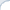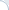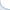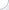English»Software»Data Structures & Algorithms»Java Software for Linear Algebra | searchivarius.orgApache Commons Library for Linear Algebra COLT   - a set of Open Source Libraries for High Performance Scientific and Technical Computing written in Java and developed at CERN (including Linear Algebra). CSparseJ   - a Java port of CSparse (a Concise Sparse matrix package) Efficient Java Matrix Library (EJML)   - a linear algebra library for manipulating dense matrices. JBLAS   - Linear Algebra for Java (dense matrices): a light-wight wrapper around the BLAS and LAPACK routines. Note that it requires native libraries of the LAPACK and BLAS routines. la4j   - linear algebra for Java (both sparse and dense matrices) Matrix Toolkits Java (MTJ)   - a high-performance library for developing linear algebra applications; based on BLAS and LAPACK for its dense and structured sparse computations. Parallel COLT   - a multi-threaded version of COLT